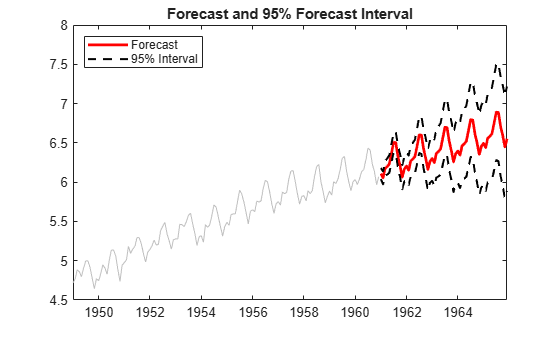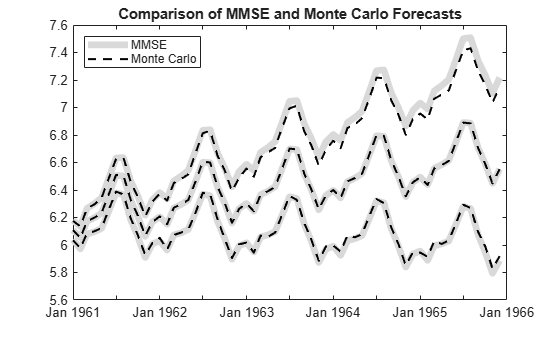# Forecast Multiplicative ARIMA Model

This example shows how to forecast a multiplicative seasonal ARIMA model using `forecast`. The time series is monthly international airline passenger numbers from 1949 to 1960.

### Load the Data and Estimate a Model.

Load the data set `Data_Airline`.

```load('Data_Airline.mat') y = log(Data); T = length(y); Mdl = arima('Constant',0,'D',1,'Seasonality',12,... 'MALags',1,'SMALags',12); EstMdl = estimate(Mdl,y);```
``` ARIMA(0,1,1) Model Seasonally Integrated with Seasonal MA(12) (Gaussian Distribution): Value StandardError TStatistic PValue _________ _____________ __________ __________ Constant 0 0 NaN NaN MA{1} -0.37716 0.066794 -5.6466 1.6364e-08 SMA{12} -0.57238 0.085439 -6.6992 2.0952e-11 Variance 0.0012634 0.00012395 10.193 2.1406e-24 ```

### Forecast Airline Passenger Counts.

Use the fitted model to generate MMSE forecasts and corresponding mean square errors over a 60-month (5-year) horizon. Use the observed series as presample data. By default, `forecast` infers presample innovations using the specified model and observations.

```[yF,yMSE] = forecast(EstMdl,60,y); upper = yF + 1.96*sqrt(yMSE); lower = yF - 1.96*sqrt(yMSE); figure plot(y,'Color',[.75,.75,.75]) hold on h1 = plot(T+1:T+60,yF,'r','LineWidth',2); h2 = plot(T+1:T+60,upper,'k--','LineWidth',1.5); plot(T+1:T+60,lower,'k--','LineWidth',1.5) xlim([0,T+60]) title('Forecast and 95% Forecast Interval') legend([h1,h2],'Forecast','95% Interval','Location','NorthWest') hold off```The MMSE forecast shows airline passenger counts continuing to grow over the forecast horizon. The confidence bounds show that a decline in passenger counts is plausible, however. Because this is a nonstationary process, the width of the forecast intervals grows over time.

### Compare MMSE and Monte Carlo Forecasts.

Simulate 500 sample paths over the same forecast horizon. Compare the simulation mean to the MMSE forecast.

```rng 'default'; res = infer(EstMdl,y); Ysim = simulate(EstMdl,60,'NumPaths',500,'Y0',y,'E0',res); yBar = mean(Ysim,2); simU = prctile(Ysim,97.5,2); simL = prctile(Ysim,2.5,2); figure h1 = plot(yF,'Color',[.85,.85,.85],'LineWidth',5); hold on h2 = plot(yBar,'k--','LineWidth',1.5); xlim([0,60]) plot([upper,lower],'Color',[.85,.85,.85],'LineWidth',5) plot([simU,simL],'k--','LineWidth',1.5) title('Comparison of MMSE and Monte Carlo Forecasts') legend([h1,h2],'MMSE','Monte Carlo','Location','NorthWest') hold off```The MMSE forecast and simulation mean are virtually indistinguishable. There are slight discrepancies between the theoretical 95% forecast intervals and the simulation-based 95% forecast intervals.